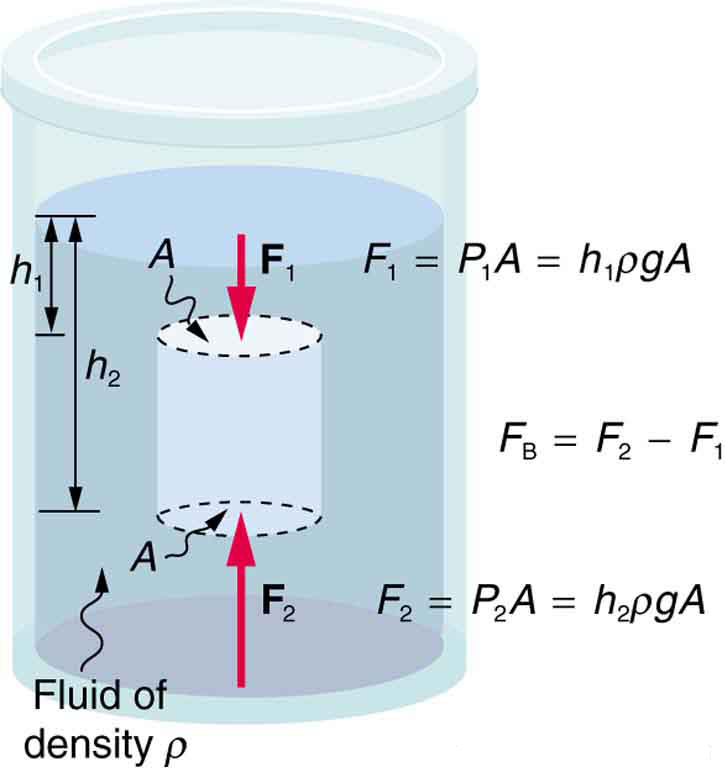# Archimede’s Principle

• When a solid object is immersed in a fluid, an upward force acts on it.
• The magnitude of the force is given by Archimede’s principle.
• This principle was propounded by Archimedes (287- 212 BC), a famous Greek scientist.
• Archimede’s principle states , “ When a body is partially or wholly immersed in a fluid, it experiences an up-thrust which is equal to the weight of the fluid displaced by it.”

Theoretical proof of Archimede’s principle

Let us consider a cylinder of height ‘h’ and uniform cross-section area ‘A’ is immersed in a liquid of density ‘d’.If h1 and h2 are the depths of the upper and lower surfaces of the immersed cylinder,  then

Force on upper face (F1) = P1 × A =h1dgA

Force on lower face (F2) = P2×A =h2dgA [g =acceleration due to gravity]

Now, Up-thrust (U) =F2 –F1

= h2dgA –h1dgA

Let h2 –h1 =h, then,